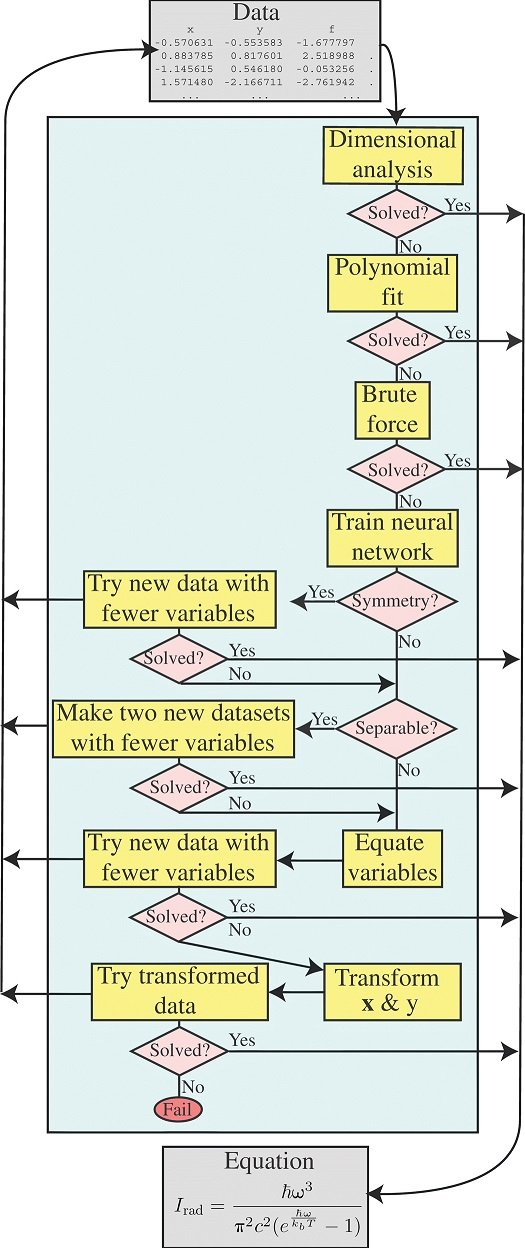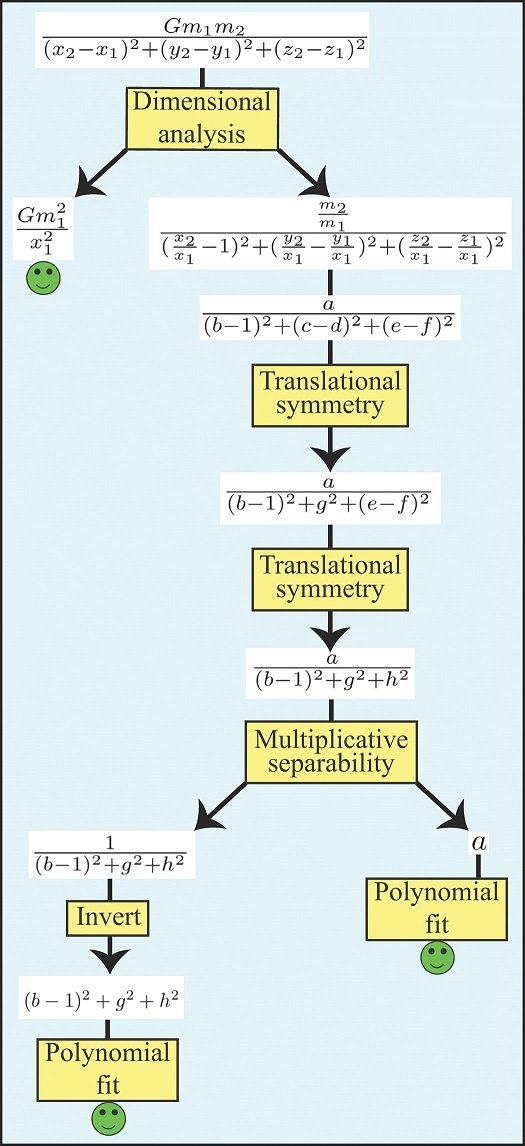# Catch up on the latest AI articles# Discovering The Feynman Physics Equations With Machine Learning: AI Feynman

3 main points
✔️ Solve the problem by repeatedly transforming it to something simpler and with fewer variables
✔️ Improve function identification problems with neural networks
✔️ Achieve prediction accuracy that outperforms existing software

written by Silviu-Marian Udrescu (MIT), Max Tegmark (MIT)
(Submitted on 27 May 2019 (v1), last revised 15 Apr 2020 (this version, v2))

Subjects: Computational Physics (physics.comp-ph); Artificial Intelligence (cs.AI); Machine Learning (cs.LG); High Energy Physics - Theory (hep-th)code：The images used in this article are from the paper or created based on it.

## first of all

In 1601, Kepler tried to explain the orbit of Mars from data, and after 40 failed attempts, he discovered that the orbit was elliptical. This is an example of symbolic regression, where a symbolic expression is found that matches specific data.

The simplest symbolic regression is a linear regression with linear combination, but the general symbolic regression problem remains unsolved. The reason for this is that when a function is encoded as a string of symbols, it grows exponentially with the length of the string, making it computationally infeasible to test all strings.

Genetic Algorithms are a method for solving problems in such a large search space and are implemented in the Eureqa software, which has been successful in symbolic regression problems.

In this research, we further improve on this state-of-the-art with neural networks. We have discovered hidden simplicity, such as symmetry and separability of mystery data, and recursively split difficult problems into simpler problems with fewer variables.

## algorithm

The general function f is very complex and almost impossible to discover by symbolic regression. However, functions that appear in physics and many other sciences often have to simplify properties that make them easier to discover, such as

(1) Units
The function f and the variables on which it depends have known physical units. This makes dimensional analysis possible, and it is sometimes possible to transform a problem into a simple problem with few independent variables.

(2) low-degree polynomials
f (or part of it) is a low-degree polynomial. This allows you to solve simultaneous equations to determine the polynomial coefficients and solve the problem quickly.

(3) Monotonicity
f is a small composition of basic functions, each of which usually takes no more than two arguments.　This allows us to represent f as an analysis tree with a small number of node types.

(4) Smoothness.
f is continuous and possibly analytic. This makes it possible to approximate f using a neural network.

(5) Symmetry
f denotes the symmetry of translation, rotation, and scaling concerning its variables. The problem can be transformed into a simple problem with less than one independent variable.

(6) Divisibility
f can be written as the sum or product of two parts that have no variables in common. We can divide the independent variables into sets that are elementary to each other and transform the problem into two simple sets.

The algorithm is shown in Fig.This is what human scientists do, they take different strategies one at a time, and if they can't solve the problem at once, they try to break it up into separate parts.

The following diagram shows an example of how Newton's law of gravity with nine variables can be solved.### dimensional analysis

The dimensional analysis module takes advantage of the fact that many problems in physics can be simplified under the assumption that the units on both sides of an equation coincide. This often transforms a problem into a simple problem with a small number of variables that are all dimensionless.

### polynomial fit

The proposed method uses standard methods for solving simultaneous linear equations to find the optimal polynomial coefficients.

### Brute force

Brute force symbolic regression models can in principle solve for all unknown functions, but in most cases, this is not practical from a computational point of view. Once the unknown data has been transformed/decomposed into simpler parts, the Brute force method is usually the most useful.

### Neural Network Based Testing

Even after applying dimensional analysis, much of the data is too complex to be solved by polynomial fitting or Brute force in a reasonable amount of time. For example, to test if there is a parallel shift in the function f, we need to test f(x1,x2) = f(x1+a,x2+a), but usually the data set does not have an interval for that variable, and thus accurate high-dimensional interpolation between data points is required to perform the test.

To obtain such an interpolation function, we train the neural network to predict the output given the input.

This may be easier than in many other contexts because neural networks do not care whether they are poorly generalized outside some domain of data. As long as it is very accurate within this domain, it will serve the purpose of correctly factorizing separable functions.

### database

To enable quantitative testing of this and other symbolic regression algorithms, a 6 gigabyte Feynman Symbolic Regression Database (FSReD) has been created and is available at https://space.mit.edu/home/tegmark/aifeynman.html and made available for free download from

To sample equations from a wide range of physics disciplines, a database has been generated using 100 equations from the seminal Feynman Lectures on Physics. In addition to this, 20 more challenging equations have been taken from other original physics books. We refer to these as Bonus mysteries.

## Results Comparison

Both the AI Feynman and Eureqa algorithms were applied to the Feynman database to perform symbolic regression and compared using up to 2 hours of CPU time per data. The results showed that Eureqa solved 71% of the data, while AI Feynman solved 100% of the data.

A closer examination of the results shows that the problems that Eureqa was unable to solve were for the most complex data.

Neural networks are even more important when rerunning AI Feynman without the dimensional analysis module because neural networks can eliminate variables by discovering symmetry and separability. The neural network solves 93% of the Feynman equation by finding separability and symmetry.

AI Feynman iteratively reduces the number of independent variables as the process progresses, virtually guaranteeing that it is moving in the right direction. In contrast, genetic algorithms such as Eureqa make progress by successively finding better approximations, but there is no guarantee that the more accurate symbolic representation is close to the truth.

If Eureqa finds a fairly approximate but inexact expression in a completely different functional form, there is a risk that it will fall into that local solution. This reflects a fundamental challenge of the genetic approach.

### Data Size Impact

To investigate the impact of changing the size of the datasets, we repeatedly reduced the size of each dataset by a factor of 10 until AI Feynman failed. Most equations are detected by polynomial fitting and Brute force, even when only 10 data points are used.

If the true equation is complex, the algorithm may overfit and "find" the wrong equation, which in some cases requires 100 data points.

Equations that need to be solved using a neural network require a significant number of data points (100 to 1000000) so that the network can learn the unknown function accurately enough.

### Effect of noise

We added a normal random number to its dependent variable y because in most cases actual data has measurement errors and other noise.

Increase the noise level from 0.000001 until AI Feynman fails. Most equations can be recovered accurately with values below 0.0001, but half of them can be solved with 0.01.

### Bonus mysteries

The previous 100 equations should be regarded as a training set because we improved the implementation and hyperparameters to optimize the performance of the AI Feynman algorithm. On the other hand, the 20 Bonus mysteries can be considered as a test set because they were analyzed after the AI Feynman algorithm and its hyperparameters were set. While Eureqa solved only 15% of the Bonus mysteries, AI Feynman solved 90% of them.

The fact that success rates vary widely in bonus mysteries reflects the increasing complexity of the equation and the need for neural network-based strategies.

## summary

The neural network strategy was able to solve the most difficult equations while Eureqa's genetic algorithm could not.

The genetic algorithm can output not only one expression, but also several possible expressions. It is not clear which one is correct, but it is more likely that one of them is the correct formula. Similarly, you can upgrade Brute force to return a candidate solution instead of a single formula.

The proposed method can also be directly integrated into larger programs that find equations involving derivatives and integrals, which occur frequently in physical equations. It is also an important idea that combining the capabilities of both the present method and the genetic algorithm may yield a method that is better than both.

If you have any suggestions for improvement of the content of the article,